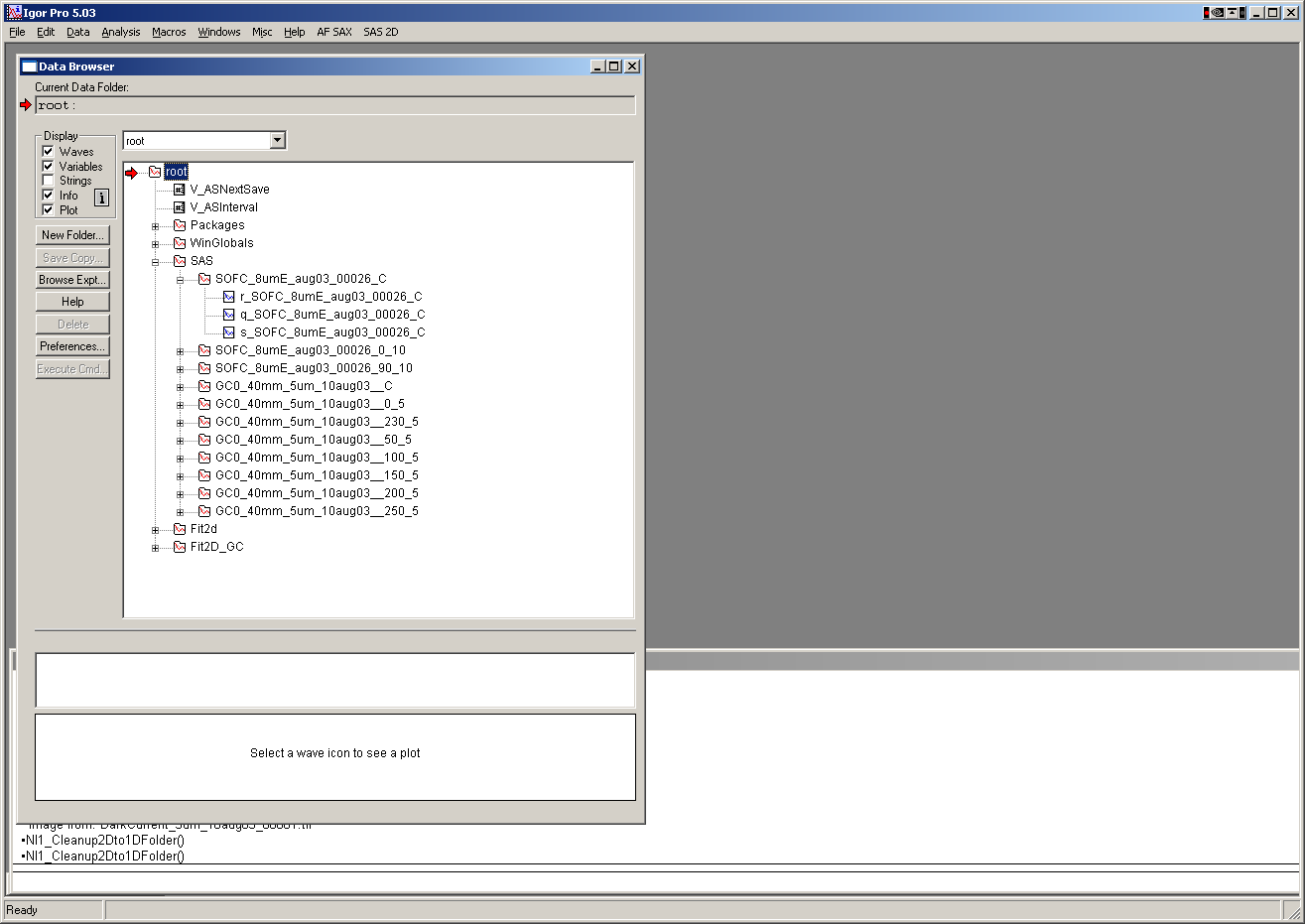# Output data¶

## QRS - Internal in Igor¶Data are internally stored (if selected) within Igor experiment in folder root:SAS: in folders with

nameOfSample_C being the circular average

nameOfSample_Angle_halfWidth being sector average around direction Angle with sector half-width.

The wave names

X-axis data

q_NameOfSample_C (or _Angle_halfWidth) q vector in A:sup:-1

t_ NameOfSample_C (or _Angle_halfWidth) 2 theta, if output with respect to 2 theta

d_ NameOfSample_C (or _Angle_halfWidth) d for output wrt d

y axis data

r_ NameOfSample_C (or _Angle_halfWidth) intensity (if calibrated in whatever units – thickness is converted to cm, so it should be cm:sup:-1)

error

s_ NameOfSample_C (or _Angle_halfWidth) error for intensity

other

w_ NameOfSample_C (or _Angle_halfWidth) width of each bin of Q/d.2 theta. This is for LUT output, and provides data for bin-width smearing. Smaller number of bins, larger width of each. For linear binning, this is same number and is (Max-Min/numOfPoits), but for log binning this is varying function of bin position.

For Line profile data:

For example for GI_Vertical line in my test case, this was the name:

gc_saxs_395__GI_VLp_0.0077

“gc_saxs_395_”…. Part of the name of used image

GI_VLp_…. GI_Vertical Line

0.0077 …. q:sub:y value at which the data were calculated.

Exported data are Int, error, Q, qx, qy, qz columns with header and column names

Saved data in Igor are

r_NameOfSample_ProfileIndicator_Qvalue intensity

q_NameOfSample_ProfileIndicator_Qvalue q [A:sup:-1]

s_NameOfSample_ProfileIndicator_Qvalue error

qy_NameOfSample_ProfileIndicator_Qvalue qy [A:sup:-1]

qz_NameOfSample_ProfileIndicator_Qvalue qz [A:sup:-1]

qx_NameOfSample_ProfileIndicator_Qvalue qx [A:sup:-1] (generated ONLY if GI… profile is used)

Note, intensity wave has attached wave note, containing some useful information: